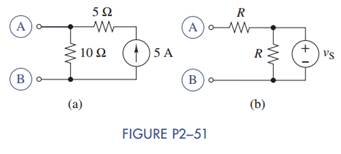### Create an Account

Home / Questions / For each of the circuits in Figure P2−51 find the equivalent practical voltage source at t...

# For each of the circuits in Figure P2−51 find the equivalent practical voltage source at terminals A and B

For each of the circuits in Figure P2−51, find the equivalent practical voltage source at terminals A and B.Jun 15 2020 View more View LessSubscribe To Get Solution